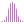#LinRegVariance (MDX)

Calculates the linear regression of a set, and returns the variance associated with the regression line, y = ax + b.

#### Syntax

LinRegVariance( <List> , , )